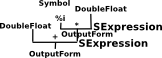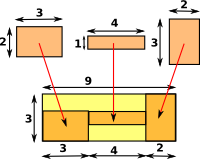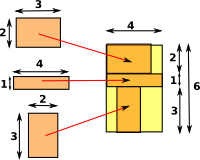# Monospace Formatter

For a more general discussion of the principles of output formatters see page here.

On this page we specifically discuss a formatter for outputting text to a monospaced, two-dimensional output device, typically the command line console.

The existing mathprint and mathprintWithNumber in 'i-output.boot' (sometimes known in the documentation as algebraFormat) is written in boot code and this is intended to have similar functionality using SPAD code.

I have put the code for the work that I have done, so far, for this here.

## Overview

Each character has a fixed width, so we can represent a mathematical expression by a rectangular array of characters, like this:

Note the use of spaces to make sure everything is aligned, this only works if every character (including spaces) has the same width.

 y x = - - - 2This program takes in the expression as a tree structure, like the one on the left.
This is converted to the rectangular array of characters, like this:
 2 + 3 % i

In the code, here, I have a domain 'CharRectangle' which represents these character arrays and has functions for constructing them, for instance:

#### hconcat:(a:List %) -> %

In this case we might have the strings "2", "+" and "3%i" and we want them to appear next to each other. In this case we use hconcat for horizontal concatenation.
 2 + 3 % iIn general hconcat can combine rectangles, of any dimension, and they will be padded out to fit into a new rectangle as required. This is done by calculating the maximum height of all the rectangles and then padding them all out to that maximum height, they can then be joined side by side.

#### vconcat:(a:List %) -> %

In this case we might have the strings "y", "---" and "2i" and we want them to appear above each other. In this case we use vconcat for vertical concatenation.

 y - - - 2In general vconcat can combine rectangles of any dimension and they will be padded out to fit into a new rectangle as required. This is done by calculating the maximum width of all the rectangles and then padding them all out to that maximum width, they can then be joined on top of each other.

#### aconcat:(a:List List %) -> %This concatenates an array of rectangles. Building an array of rectangles can't always be done by doing vconcat first and then hconcat, or the other way round, because they will be aligned vertically but not horizontally or aligned horizontally but not vertically. So we need this when we want to align in both dimensions simultaneously. So in this example on the left, the rectangles at the top are put in a big rectangle at the bottom. The way to understand this is to imagine the rectangles being put into a grid. This is done by calculating the maximum widths of all the columns [4,4,3] and all the rows [3,2,3]. We can then slot each rectangle into its place in the grid.

#### Examples are:

a^2

 2 a

matrix([[1,2],[3,4]])

 + 1 2 + | | + 3 4 +

## Interim Approach to Trying the Code

I am currently testing by compiling with the same name as an existing formatter: 'htmlFormat'. So the output can then be enabled by typing:

`)set output html on`

## Installing

If you don't want to replace the html formatter but want to add it as an additional formatter then the following files need to be modified:

• src/interp/setvars.boot.pamphlet
• src/algebra/Makefile.pamphlet
• src/algebra/exposed.lsp.pamphlet
• src/doc/axiom.bib.pamphlet
• interp/i-output.boot.pamphlet

## Using the formatter

We can cause the command line interpreter to output by typing the following:

`)set output monospace on`

After this the command line will output (in addition to other formats that are enabled).

## Line Folding

As it stands I think the code here does a reasonable job of displaying all the various types of expressions in FriCAS, although it is not perfect. It does not yet handle line wrapping very well, the program currently generates an array of Character and then outputs this array to the output device. I could easily check the width of this array and, if greater than the line width, choose a place to divide it that does not break apart numbers or names.

## Programmers Guide

This package converts from OutputForm, which is a hierarchical tree structure,
to an array of Character.

This is created by traversing OutputForm while building up an array of
Character which represents a rectangular area of screen.

The second stage is to output this Character rectangle.